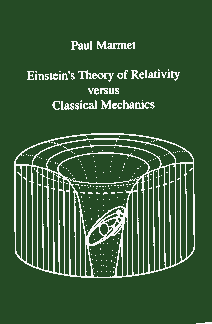Enter the content which will be displayed in sticky bar

# BookPages: 200
Publisher: Newton Physics Books
Year: 1997
ISBN: 0921272189
ISBN: 978-0921272182

Einsteins Theory of Relativity Versus Classical Mechanics (Buy Now)
KeyWords: einstein

The aim of this book is to demonstrate that using "Conventional Wisdom" and "Conventional Logic", classical physics can explain all the observed phenomena attributed to relativity. The arbitrary principles of Einstein's relativity are thus useless.

It is very important to recognize the fundamental importance of the principle of mass-energy conservation. It took thousands of years of development for scientific thought to finally reject the magic of witchcraft. During the nineteenth century, scientists became convinced that matter cannot be created from nothing. Conversely, matter cannot be destroyed into nothing. It seems that even Einstein believed this, since he is the one who, at the beginning of the twentieth century, introduced the equation E = mc2 implying mass-energy conservation. However, he later developed general relativity which is not compatible with that principle. Indeed, according to Straumann, the "general conservation law of energy and momentum does not exist in general relativity".  Twentieth century science moved backward in accepting again the magical creation of matter or energy from nothing, even if this is hidden in complicated mathematics.

Contrary to what Einstein did, all the demonstrations in this book are compatible with the principle of mass-energy and momentum conservation. Using classical mechanics, we demonstrate that length contraction is a real physical phenomenon. We examine how this leads to the Lorentz equations. Then, we show how classical principles are sufficient to explain the advance of the perihelion of Mercury and derive Einstein's equation. The fundamental reason for this advance is illustrated with a classical apparatus. We also study the Lorentz transformations in three dimensions and the Doppler phenomenon. Then we see how the problems brought by the relativity of simultaneity and by the principle of equivalence can be explained using conventional logic. We also show how classical mechanisms produce perturbations in the internal structure of atoms and molecules. Finally, we show that the presence of intense gravitational potentials leads to degenerate matter corresponding to Schwarzschild's black holes.

Einstein's relativity principles are not needed in these demonstrations. The only principles used are the ones already existing in classical mechanics. All the solutions are based on a physical model compatible with conventional logic.

Einstein's theory of relativity is a mathematical model which is not compatible with the physical models described in classical mechanics since it is not compatible with the principle of mass-energy conservation. This is a well-known fact. It is claimed that the theory of relativity is so advanced that it is not possible to give a Newtonian physical description of it. It is also often argued that abandoning classical scientific concepts leads to a scientific revolution. It is erroneous to believe that a new scientific revolution must abandon the fundamental principles brought up by Newton's classical mechanics and logic which gave birth to all our knowledge in physics.
As stated in several papers, Einstein's relativity implies "New Logic" which contradicts "Conventional Logic". Einstein's theory implies that because we can find some arbitrary mathematical relationships that fit some experiments, we must abandon conventional logic. History reports some rudimentary scientific models that also fitted experiments but which were based on nonsense. Those models were rejected. A new scientific revolution based on "New Non Conventional Logic" can lead to a scientific disaster or to a dead end. No scientific concept can be so advanced that it is no longer compatible with logic.

Einstein's relativity assumes new mathematical hypotheses and ignores completely the concept of models to describe physical reality. Einstein supposed that time and space can be distorted and that simultaneity is relative but he did not give any serious description of what this really means physically. In Newton's time, physical descriptions of phenomena were accompanied by mathematical equations giving quantitative predictions corresponding to those physical descriptions. Einstein's relativity claims that nature can be described with mathematical equations without any physical description. There is a complete abandon of all the physical models that made physics understandable in Newton's time.

Our main argument here is not whether Einstein's hypotheses are true or not. We believe that if Einstein's hypotheses are correct, they must correspond to a real physical mechanism. Such a real mechanism is described in this book using classical mechanics and classical logic.

With Einstein's new logic, contradictory results have appeared. For example, Gerald Feinberg developed the theory of tachyons which move faster than the speed of light. There are also mathematical models calculating wormholes, strings, multidimensional space, superluminal objects, time reversal and even time lines. Certainly, these claims do not make sense when we use conventional logic.

An expert in Einstein's relativity is described as an expert in the mathematics of relativity. Since the conventional wisdom of classical physics is not used in relativity, an expert in relativity is not trained to deal with Newtonian logic. Consequently, this book on relativity will be much more easily understood by an expert in classical physics since he or she already knows the mathematics and understands the classical mechanisms involved. It might appear surprising to some readers that relativity can be explained with classical principles. However, they will never escape out of their preconceived notions and learn how this is done unless they carefully read this book. - From the Preface.